Chapter 13

From
our electron model, we find out that the electromagnetic field has satisfied the 5 equations (4.1), (5.1), (10.7), (11.7), (12.7), here we rewrite each one of the equations as follows:

The Gauss Law of Electric Field: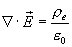(13.1)

The Gauss Law of Magnetic Field: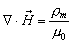(13.2)

The velocity of electromagnetic field equation: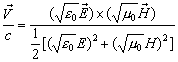(13.3)

The hydroelectric equation: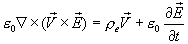(13.4)

The hydromagnetic equation: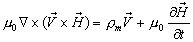(13.5)

Let us consider one special case based on the above 5 electromagnetic field equations, the free charge electromagnetic field in vacuum, which satisfies the following four conditions: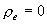(13.6)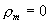(13.7)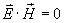(13.8)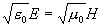(13.9)

Then, based on above 4 conditions, we get: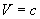(13.10)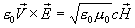(13.11)

Thus: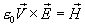(13.12)

And: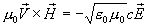(13.13)

Thus: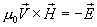(13.14)

Thus, we can get the following 5 equations of the electromagnetic field in vacuum of free charge, which are: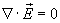(13.15)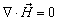(13.16)

V=c                                                                                                                       (13.17)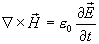(13.18)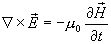(13.19)

Then based on the above 5 equations, we can get the Maxwell Electromagnetic Wave Equation in vacuum of free charge: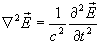(13.20)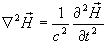(13.21)